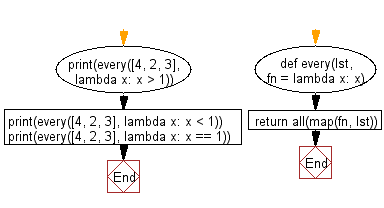﻿ Python: Check if a given function returns True for every element in a list - w3resource# Python: Check if a given function returns True for every element in a list

## Python List: Exercise - 243 with Solution

Write a Python program to check if a given function returns True for every element in a list.

Use all() in combination with map() and fn to check if fn returns True for all elements in the list.

Sample Solution:

Python Code:

``````def every(lst, fn = lambda x: x):
return all(map(fn, lst))
print(every([4, 2, 3], lambda x: x > 1))
print(every([4, 2, 3], lambda x: x < 1))
print(every([4, 2, 3], lambda x: x == 1))
```
```

Sample Output:

```True
False
False
```

Flowchart:## Visualize Python code execution:

The following tool visualize what the computer is doing step-by-step as it executes the said program:

Python Code Editor:

Have another way to solve this solution? Contribute your code (and comments) through Disqus.

What is the difficulty level of this exercise?

Test your Python skills with w3resource's quiz

﻿

## Python: Tips of the Day

Floor Division:

When we speak of division we normally mean (/) float division operator, this will give a precise result in float format with decimals.

For a rounded integer result there is (//) floor division operator in Python. Floor division will only give integer results that are round numbers.

```print(1000 // 300)
print(1000 / 300)```

Output:

```3
3.3333333333333335```